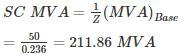Courses

# Power Systems - 7

## 10 Questions MCQ Test GATE Electrical Engineering (EE) 2022 Mock Test Series | Power Systems - 7

Description
This mock test of Power Systems - 7 for Electrical Engineering (EE) helps you for every Electrical Engineering (EE) entrance exam. This contains 10 Multiple Choice Questions for Electrical Engineering (EE) Power Systems - 7 (mcq) to study with solutions a complete question bank. The solved questions answers in this Power Systems - 7 quiz give you a good mix of easy questions and tough questions. Electrical Engineering (EE) students definitely take this Power Systems - 7 exercise for a better result in the exam. You can find other Power Systems - 7 extra questions, long questions & short questions for Electrical Engineering (EE) on EduRev as well by searching above.
QUESTION: 1

### Determine the fault current in a system following a double line to ground short circuit at the terminals of a star connected synchronous generator operating initially on an open circuit voltage of 1.0 pu. The positive, negative and zero sequences reactance of the generator are, respectively j 0.35, j 0.25 and j 0.20 and the star point is isolated from ground.

Solution:

Since the star point is isolated from ground LLG fault is just like LL fault.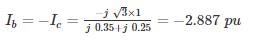*Answer can only contain numeric values
QUESTION: 2

### A 50 Hz overhead Line has Line to each capacitance of 2 μF. It is decided to use an earth fault neutralizer. The reactance to neutralizer. The reactance to neutralize the capacitance of 80% of the length of the line is – (in ohms)

Solution: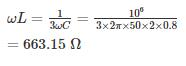QUESTION: 3

### A positive, negative and zero sequence impedance of 3ϕ generator are Z1, Z2 and Z0 respectively. For a line to line fault with fault impedance ZF, the fault current is IF1 = KIF. Where IF is the fault current with zero fault impedance. The relation between ZF and K is

Solution: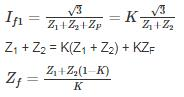*Answer can only contain numeric values
QUESTION: 4

A three phase 15 MVA, 13 kV alternator has an initial reactance of 5%. The series reactor needed to restrict the short circuit current of the alternator to 10 times of full load current is _____ (in Ω).

Solution:

Short circuit current ISC =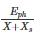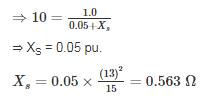QUESTION: 5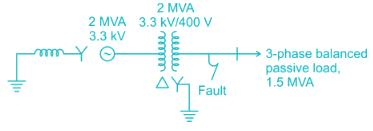A 2 MVA, 3.3 kV, three-phase generator as the following reactance’s on its own base:

+ve sequence reactance, x1 = j0.10 p.u.

-ve sequence reactance, x2 = j0.10 p.u.

Zero sequence reactance, x0 = j0.05 p.u.

Reactance from neutral to ground, xn = j0.05 p.u.

The generator supplies a 3-phase, 1.5 MVA balanced passive load through a 3-phase, 2.0 MVA, 3.3 kV/400 V, delta-star transform with leakage reactance of 10%, as shown in the figure. A single-line-to-ground fault takes place at the low voltage side of the transformer. The current flowing from the transformer to the fault is (Assume base MVA is 1.5 MVA)

Solution:

The generator supplies a 3-phase, 1.5 MVA balanced passive load.

so, the system works under a base load of 1.5 MVA

The generator reactance on a base load of 1.5 MVA are as follows.
+ve sequence reactance x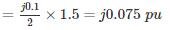-ve sequence reactance x=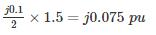zero sequence reactance x0 =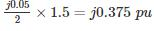Reactance form neutral to ground xn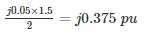leakage reactance of transformer x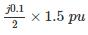= j0.075 pu

Positive sequence network for the above system is,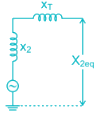X1eq = x+ xT

= j0.075 + j0.075

= j0.15 pu

Negative sequence network for above system is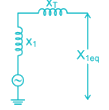X2eq = x2 + xT = j0.075 + j0.075

= j0.15 pu

Zero sequence network for above system isX0eq = xT = j 0.075 pu

For a line to ground fault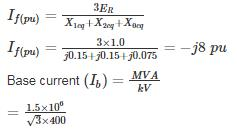= 2165.06 A

Fault current (If) = (Ib) × (If(pu))

= 2165.06 × 8

= 17320.5 A

*Answer can only contain numeric values
QUESTION: 6

A 10 MVA, 6.6 kV generator has percentage reactances to positive, negative and zero sequence currents of 15%, 10%, and 5% respectively and it's neutral is solidly grounded. The generator is unloaded and excited to its rated voltage. The fault current (in kA) will be when a single line to ground fault develops on phase a of the generator. The phase sequence is ABC.

Solution:

On base MVA of 10 and base voltage of 6.6 kV

Z1 = j 0.15 pu

Z2 j 0.10 pu

Z0 = j 0.05 pu

Zn = 0, Zf = 0

For L-G fault on phase ‘a’

Ib = Ic = 0 and Va = 0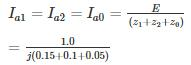= -j 3.33 pu

Fault current, If = Ia = 3 Ia1 = -j 10 pu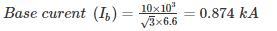Fault current = Ib × If (pu)

= 8.74 kA

*Answer can only contain numeric values
QUESTION: 7

For an unloaded generator, at rated voltage, the magnitude of fault current for a three-phase fault is the same as LG fault. The reactances of the generator are

x1 = 0.2 pu, x2 = 0.15 pu, x0 = 0.05 pu

The value of the neutral grounding reactance is ______ (in pu)

Solution:

x1 = 0.2 pu, x2 = 0.15 pu, x0 = 0.05 pu

Magnitude of fault current for three phase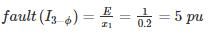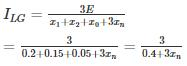Given that, I3-ϕ­ = ILG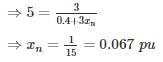QUESTION: 8

The severity of line to ground and three phase faults is to be same. If the terminal voltage is 1.0 pu, Z1 = Z2 = j 0.1 pu and Z0 = j 0.05 pu for an alternator, then the required inductive reactance for neutral grounding is

Solution:

Given that,

Z1 = Z2 = j 0.1 pu

Z0 = j 0.05 pu

Ea = 1 pu

For line to ground fault most server current = In = 3 Iao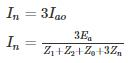For 3 - phase fault, fault current =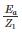As per the equation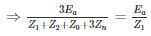⇒ 3z1 = Z1 + Z2 + Z0 + 3Zn

⇒ 3Zn = 2Z1 - Z2 - Z0

⇒ 3Zn = j2(0.1) – j0.1 – j 0.05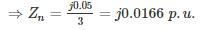*Answer can only contain numeric values
QUESTION: 9

The 33 kV busbars of a station are in two sections A and B separated by a reactor. Section A is fed from four 10 MVA generators each having a reactance of 20% section B is fed from the grid through a 50 MVA transformer of 10% reactance. The circuit breakers have each a rupturing capacity of 500 MVA. Find the reactance (in Ω) of the reactor to prevent the circuit breakers from being overloaded, if a symmetrical three-phase fault occurs on an outgoing feeder connected for it. (Take base voltage of 33 KV and base MVA of 50).

Solution:

The one-line diagram of the system is shown in figure. Given that base voltage = 33 kV

base MVA = 50 MVA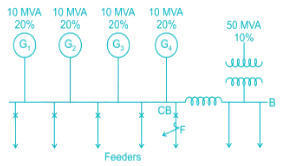Per unit reactance of generators and transformer are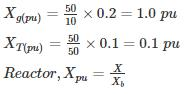For a symmetrical three-phase fault at point F on an outgoing feeder connected to A, the reactance diagram with voltage sources short circuited will be as shown below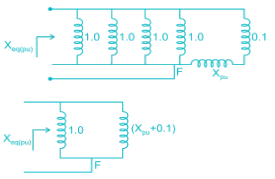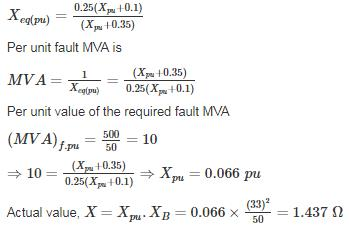QUESTION: 10

Three 6.6 kV generators A, B and C each of 10% leakage reactance and MVA ratings 40, 50 and 25, respectively are interconnected electrically as shown in the figure, by a tie bar through current limiting reactors, each of 12% reactance based upon the rating of the machine to which it is connected. A three-phase feeder is supplied from the bus bar of generator A at a line voltage of 6.6 kV. The feeder has a resistance of 0.06 Ω/phase and an indicative reactance of 0.12 Ω/phase. Estimate the maximum MVA that can be fed into asymmetrical short circuit at the far end of the feeder.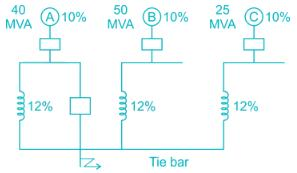Solution:

Base = 50 MVA, 6.6 kV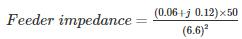= (0.069 + j 0.138) pu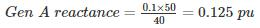Gen B reactance = 0.12 pu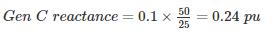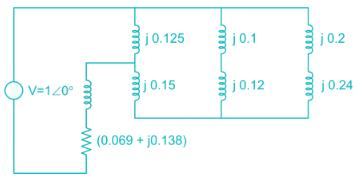Zeq = 0.069 + j 0.138 + j 0.125 [(j 0.15) + (j 0.22 || j 0.44)]

= 0.069 + j 0.226 = 0.236 ∠73°